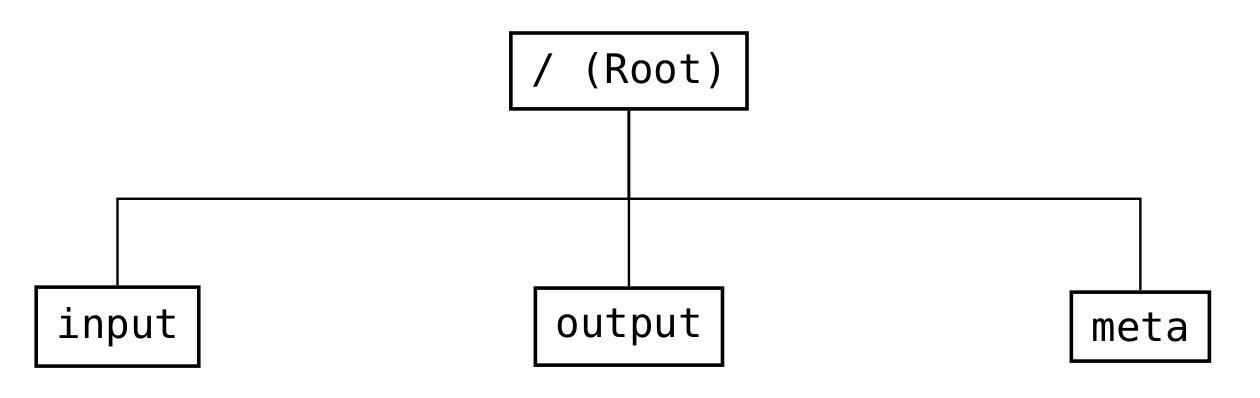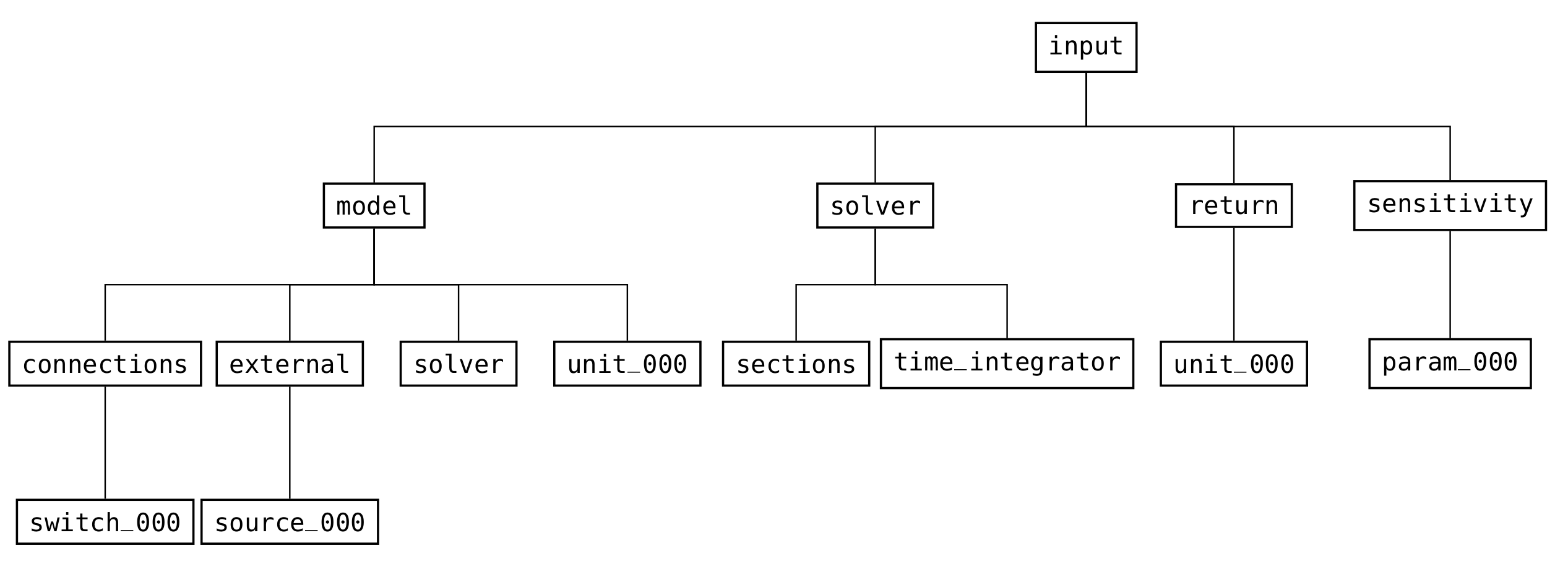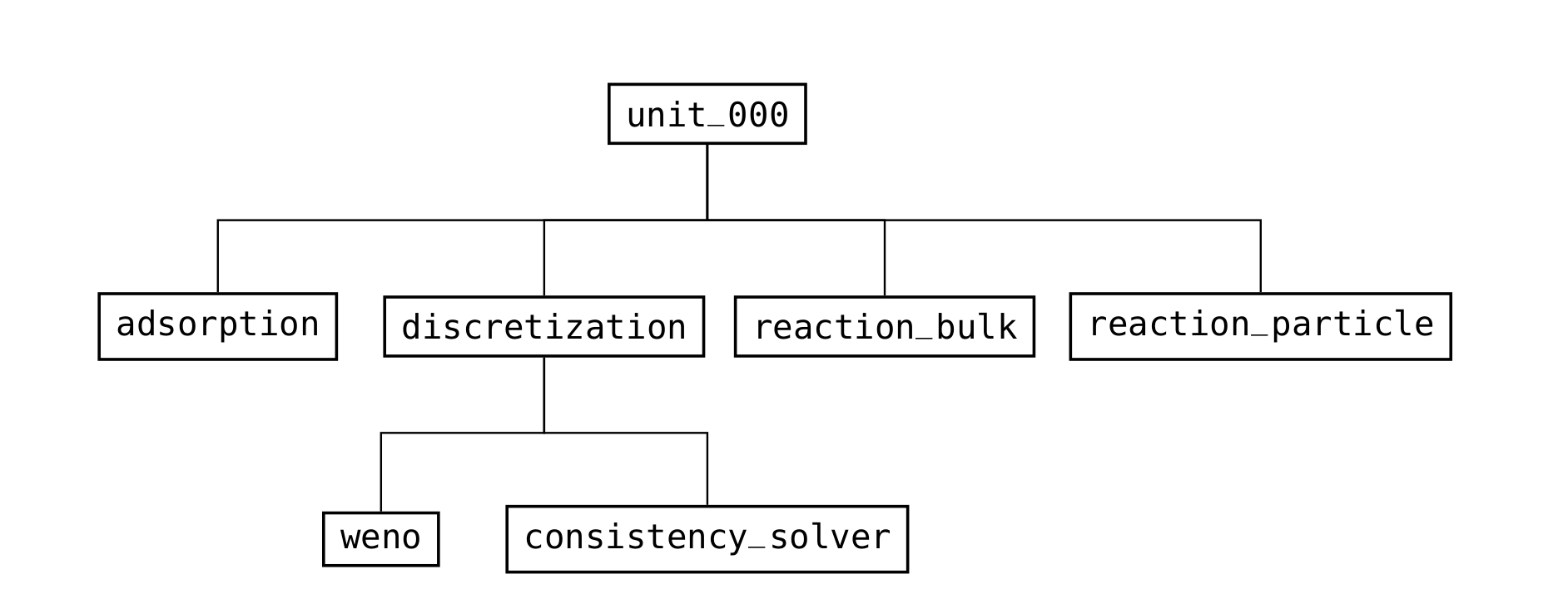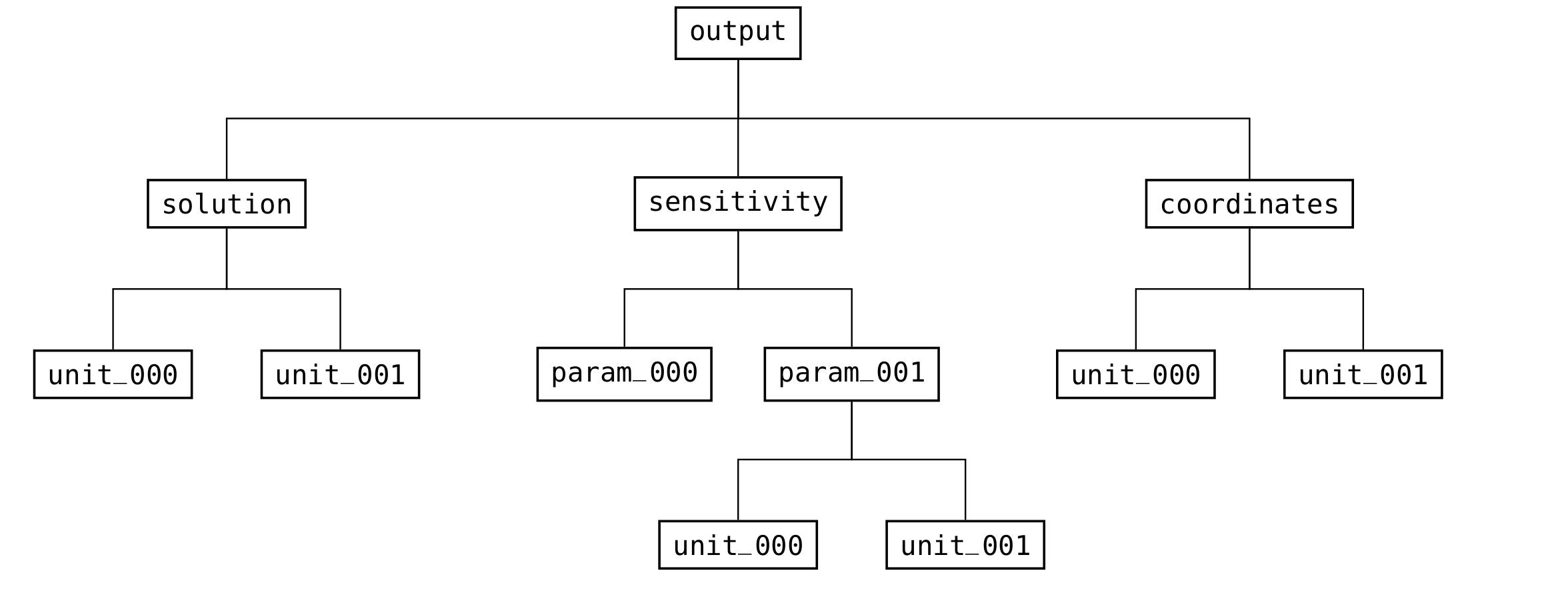You're reading the documentation for a development version. For the latest released version, please have a look at v4.3.0.

# Introduction¶

## Global structure¶

The global structure (see Fig. 7) is divided into three parts: input, output, and meta. Every valid CADET file needs an input group (see Fig. 8) which contains all relevant information for simulating a model. It does not need an output (see Fig. 10) or meta (see Fig. 7) group, since those are created when results are written. Whereas the output group is solely used as output and holds the results of the simulation, the meta group is used for input and output. Details such as file format version and simulator version are read from and written to the meta group.

If not explicitly stated otherwise, all datasets are mandatory. By convention all group names are lowercase, whereas all dataset names are uppercase. Note that this is just a description of the file format and not a detailed explanation of the meaning of the parameters. For the latter, please refer to the corresponding sections in the previous chapter.Fig. 7 Structure of the groups in the root group of the file format¶Fig. 8 High-level structure of the groups in the input part of the file format¶Fig. 9 Structure of the groups in a column unit operation (/input/model group)¶Fig. 10 Structure of the groups in the output part of the file format¶

## Notation and identifiers¶

Reference volumes are denoted by subscripts:

• $$m_{IV}^{3}$$ Interstitial volume

• $$m_{MP}^{3}$$ Bead mobile phase volume

• $$m_{SP}^{3}$$ Bead solid phase volume

Common notation and identifiers that are used in the subsequent description are listed in Table 4.

Table 4 Common notation and identifiers used in the file format description

Identifier

Meaning

NCOMP

Number of components of a unit operation

NTOTALCOMP

Total number of components in the system (sum of all unit operation components)

NPARTYPE

Number of particles types of a unit operation

NBNDi

Number of bound states of component i of the current particle type

NTOTALBND

Total number of bound states of the current particle type (sum of all bound states of all components)

NSTATES

Maximum of the number of bound states for each component of a particle type

NREACT

Number of reactions (in bulk volume or in the current particle type)

NDOF

Total number of degrees of freedom of the current unit operation model or system of unit operations

NSEC

Number of time integration sections

PARAM_VALUE

Value of a generic unspecified parameter

## Ordering of multi dimensional data¶

Some model parameters, especially in certain binding models, require multi dimensional data. Since CADET only reads one dimensional arrays, the layout of the data has to be specified (i.e., the way how the data is linearized in memory). The term “xyz-major” means that the index corresponding to xyz changes the slowest.

For instance, suppose a model with $$2$$ components and $$3$$ bound states has a “component-major” dataset. Then, the requested matrix is stored in memory such that all bound states are listed for each component (i.e., the component index changes the slowest and the bound state index the fastest):

comp0bnd0, comp0bnd1, comp0bnd2, comp1bnd0, comp1bnd1, comp1bnd2.


This linear array can be represented as a $$2 \times 3$$ matrix in “row-major” storage format, or a $$3 \times 2$$ matrix in “column-major” ordering.

## Section dependent model parameters¶

Some model parameters (see Table 5) can be assigned different values for each section. For example, the velocity a column is operated with could differ in the load, wash, and elution phases. Section dependency is recognized by specifying the appropriate number of values for the parameters (see Length column in the following tables). If a parameter depends on both the component and the section, the ordering is section-major.

For instance, the Length field of the parameter VELOCITY reads $$1 / NSEC$$ which means that it is not recognized as section dependent if only $$1$$ value (scalar) is passed. However, if NSEC many values (vector) are present, it will be treated as section dependent.

Note that all components of component dependent datasets have to be section dependent (e.g., you cannot have a section dependency on component $$2$$ only while the other components are not section dependent).

Table 5 Section dependent datasets in the 1D unit operation models (/input/model/unit_XXX group)

Dataset

Component dependent

Section dependent

COL_DISPERSION

FILM_DIFFUSION

PAR_DIFFUSION

PAR_SURDIFFUSION

VELOCITY